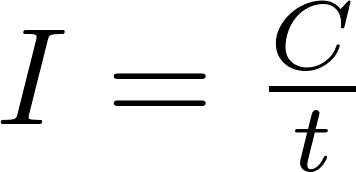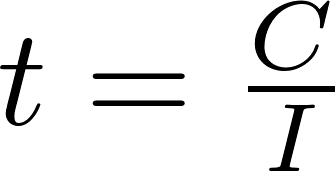# Battery discharge rate

The battery discharge rate is the speed at which the battery discharges.

### Formulas

You can use the following formulas to calculate the capacity, discharge rate and run time of a battery.t is the symbol for time and is measured in seconds (s).
C is the symbol for capacity and is measured in ampere-hour (Ah).
I is the symbol for current and is measured in ampere (A).

### Calculator

Enter two values to calculate the third.